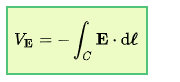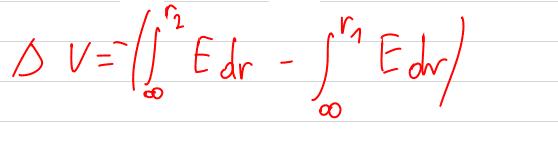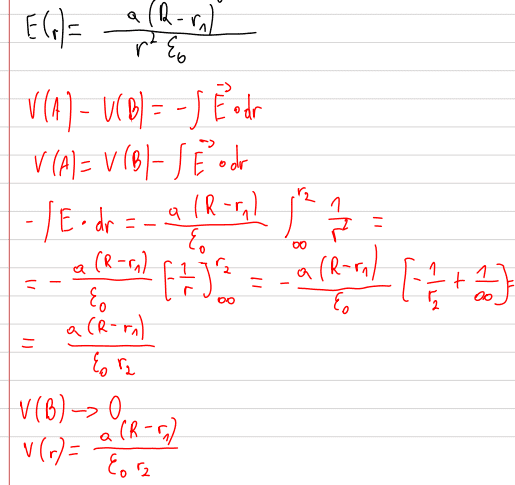# Electrostatics - electric potential and field strength (dielectric)

• polibuda
Maybe I misunderstand "qv".I don't think you are doing any integrals. You just need to use the standard results for fields inside and outside spherically symmetric distributions. All you need to figure out is the charge inside a shell of radius r.

#### polibuda

Homework Statement
The space between two concentric spheres with radii r1 and r2 (r1 <r2) is filled with a space charge with a volume density qv. Determine the dependence of the electric field strength E (r) and the potential V (r) throughout the space.
Relevant Equations
E(r), V(r)
Could somebody check my solution?

#### Attachments

•36.6 KB · Views: 118
•Delta2
It's E=-dV/dr (in a 1-D problem) and not V=dE/dr

•polibuda
Should I use this pattern? Is something else to correct in my solution?Better yet:$$V(A)-V(B)=- \int_B^A \vec E \cdot d \vec l$$

•Delta2 and polibuda
In second case the integration limits: A=r1, B=r2?
In third case the integration limits: A=r2, B=infinity?

The most common choice if you want "The Potential" is B=infinity and A=r (generic)

•polibuda
Gordianus said:
The most common choice if you want "The Potential" is B=infinity and A=r (generic)
Ok, but I need to check three cases. What with the sceond? Should it be A=r1, B=r2?

You have different functions that define E in each region. Think how to split the integral. Remember, the potential must be continuous.

Ok, in case, where r1<=r<=r2:polibuda said:
Ok, in case, where r1<=r<=r2:
View attachment 272838
You want the potential at radius r. The above expression will give the potential difference between radii r1 and r2.

First find the potential at the outer radius, then consider the potential difference between there and some smaller radius r.
For the second step, you may find it less confusing to introduce a variable of integration, s, r<s<r2.

•polibuda
haruspex said:
First find the potential at the outer radius
Do you mean r2? Wchich pattern I should use?
This one:
$$V(A)-V(B)=- \int_B^A \vec E \cdot d \vec l$$ ?
Is E equal to electric field from third case or second?
I'm sorry for my stupid questions, but I can't understand this task.

polibuda said:
Is E equal to electric field from third case or second?
What do you think? Of the radii 0, r1, r2, ∞, at which do you know the potential immediately (by common convention)?

Last edited:
$$\boxed{V(B)-V(A)=-\int_A^B\vec E\cdot d\vec s\Leftrightarrow V(B)=V(A)-\int_A^B\vec E\cdot d\vec s}$$
When you integrate ##-\vec E\cdot d\vec s##, you first find an antiderivative ##V(r)=f(r)+C##, and then you perform the usual subtraction ##V(r_B)-V(r_A)##. You can clearly see that ##C## disappears whatever its value is.

What people usually do is define ##C## such that ##\lim_{r\to\infty}V(r)=0##.
When ##r\to\infty##, which region are you in? So what's ##\vec E(r)##? Use it to find an antiderivative ##V(r)=f(r)+C##, and make ##C## verify the above condition.

•polibuda
Guys we are talking about the potential as the integral of the E-field, but I think his expressions for the E-field in cases 2 and 3 are incorrect, what do you think?

•polibuda
polibuda said:
You are sure. I forgot in task is given qv=a/r^2. I determined a new E-field in cases 2 and 3.
I think you still do the same mistake in case 2. You integrate from ##r_1## to ##r_2## in that integral but then you take your gaussian surface to be of another radius ##r##. The charge enclosed by this gaussian surface is the charge from ##r_1## to ##r## so i believe the limits of integration in the integral should be from ##r_1## to ##r##. To make a long story short, where you have ##r_2## you should replace it by ##r##. That's what i think but i might be wrong cause i haven't slept well and i can't think clearly. Better wait for the other members to share their thoughts on this.

•polibuda
polibuda said:
You are sure. I forgot in task is given qv=a/r^2. I determined a new E-field in cases 2 and 3.
I don't know why you are doing any integrals to find the fields. Just use the standard results for fields inside and outside spherically symmetric distributions. All you need to figure out is the charge inside a shell of radius r.

haruspex said:
I don't know why you are doing any integrals to find the fields. Just use the standard results for fields inside and outside spherically symmetric distributions. All you need to figure out is the charge inside a shell of radius r.
But the charge is equal to qv*volume, so I need to do integral.

•Delta2
What now with this potential? I have no r in my formula of electric potential, so I'm doing something wrong. The big R is a radius beacuse of Gauss.Last edited:
polibuda said:
But the charge is equal to qv*volume, so I need to do integral.
Maybe I misunderstand "qv". I have been taking that to be a constant charge density, as in qv. If so, what is the charge inside radius r? If not, please explain what it is.